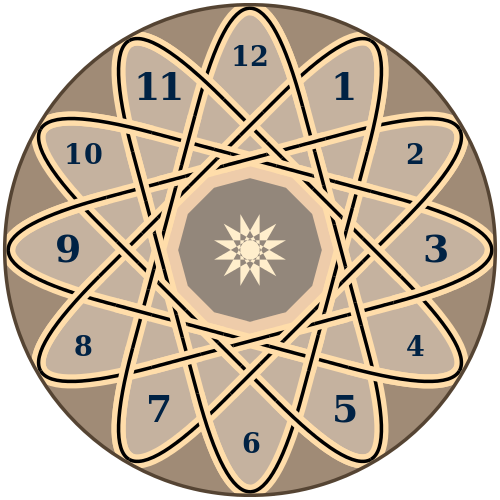# AP or No AP? Part (ii)From the set {$1,2,3,...,n$}, $11$ numbers are removed. Find the smallest $n$ such that no matter which $11$ numbers are removed, there always exists at least one Arithmetic Progression of length $12$.

×